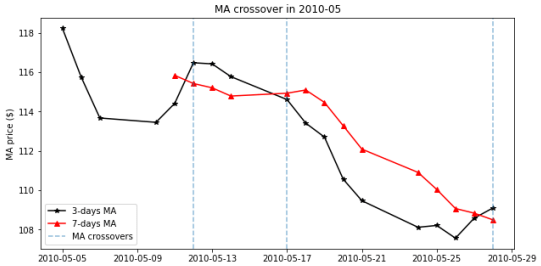# Python Programming

Question 1

An analyst wants to test the simple moving average (MA) strategy. She has a hypothesis— if a stock has many instances of long-MA and short-MA crossover in a month, it is a bullish signal. She wanted to test if her hypothesis is correct by back-testing it with historical stock prices.

Figure 1 illustrates the crossover of a long MA and a short MA. The details of calculating MA values using daily closing stock prices are given in Appendix A. In the figure, there are three (3) instances in May-2010 where the 7-days and 3-days MA crossed over. The crossovers are observed on dates denoted by the vertical blue lines.Figure 1. The crossover instances of the 7-day and 3-day MA of an unknown
stock.

The MA values are calculated using daily closing prices.
Given the following Python inputs, determine if the analyst’s hypothesis is correct.Make use of the alpha vantage module to download the prices of stock from start_dt to end_dt.